In :
import numpy as np
import pandas as pd
import matplotlib.pyplot as plt
from IPython.display import Image
%matplotlib inline

Lecture 24:¶

• Find out about Machine Learning
• Learn about using the scikit-learn python package for clustering analysis.
• Apply clustering analysis to Earth Science problems

In the next couple of lectures, we are going to learn how to use the scikit-learn package to perform some machine learning techniques.

What is machine learning anyway?¶

Machine Learning is one of those buzzwords you seem to hear all over the place these days, but what does it actually mean?

At it's simplest, machine learning is a way of finding 'features' in and assigning 'labels' to your data that allow you to build models.

There are two main types of machine learning, supervised and unsupervised.

Supervised machine learning involves labeling a subset of your data and fitting a known type of model to explain these labels. The computer then applies this model to new data and can label the new data automatically. The labels you apply to data can be a continuous set of values, known as regression or they can be discrete, known as _classification.

We're all pretty familiar with supervised machine learning already. Every time you try to log into a website and you have to click on pictures of cars or road signs to log in, you're teaching Google how to recognise those objects in images. Google then uses those data to create models that it uses to teach its self driving cars how to 'see', for example.

Unsupervised machine learning is fundamentally different because it can be applied to a dataset without training it first. This type of learning looks for features in the dataset that it can use to categorize different parts of the data (known as clustering) or define a coordinate space to help see the data, better known as (dimensionality reduction).

This all might seem quite confusing and abstract right now, but with the examples below you should start to get an idea of what you can use machine learning for. This lecture is just a small fraction of what can be done. If you want to really learn how to do it, start with this book: https://jakevdp.github.io/PythonDataScienceHandbook/ It is like this class, in that it is a bunch of Jupyter notebooks which now you are a pro at.

Today we are going to use a form of unsupervised learning (clustering) to do some geology!.

The Orocopio Mountains Dataset¶

The dataset poles_data contains a dataset of poles to bedding planes from the Orocopio mountains. We learned about poles to planes in Lecture 22. If a rock is composed of sediments that are layed down flat on top of one another, then we would expect the pole to the plane to be vertical (because the plane itself is horizontal). If instead the plane is tilted, we might expect the pole to the plane to be in some other direction. Let's peek at a data set of poles from bedding planes measured in the Orocopio Mountains.

In :
Out:
Lon Lat Pole_Az Pole_Plunge
0 -115.684573 33.547327 340.0 13.0
1 -115.684341 33.547283 359.0 14.0
2 -115.684341 33.547283 347.0 11.0
3 -115.685112 33.547408 332.0 13.0
4 -115.685650 33.547545 12.0 32.0

We can use a modified version of our equal area plot to plot the 'Pole_Az' and 'Pole Plunge' columns on an equal area projection to see what the bedding planes look like.

In :
# Here's the equal angle function

def plot_equal_area(Azs,Pls,colors='black',cmap='RdBu',alpha=1):
"""
Plots an Equal Angle plot for data d, colors are a string or list of colors
to be passed to the points in data
Note that this is different to the code in lecture 20 because it uses a
scatter plot.

Parameters:
____________
Azs : np.array
Azimuths of poles to planes
Pls : np.array
Plunges of poles to planes

Returns:
_________
None

"""
fig = plt.subplot(111, polar=True)
# set the coordinates (like for rose diagrams)
fig.set_theta_direction(-1) # Reverse direction of degrees (CW)
fig.set_theta_zero_location("N") # Specify 0-degrees as North
# for this we want the full 90 degrees, so set the scale
plt.polar(,) ## to scale grid
# plot the first direction as a red dot
# plot the second direction as a blue square

# make a list of contours to plot
# notice use of list comprehension
# label the azimuths at 20 degree intervals
AzContours=range(0,360,20)
AzLabels=[str(p) for p in AzContours]
plt.thetagrids(AzContours,AzLabels)
# and now the plunges
PlContours=[EqualArea(a) for a in range(10,90,20)] ##don't include center or edge
# make a list of labels
PlLabels=[str(a) for a in range(10,90,20)]
# draw on the plunge contours and label them
plt.rgrids(PlContours,PlLabels)
# label the plot
plt.title('Equal Area Net');
In :
plot_equal_area(poles_data.Pole_Az,poles_data.Pole_Plunge,colors='cyan')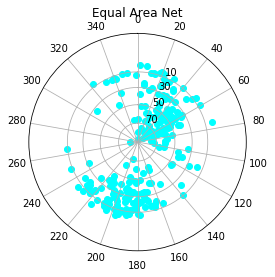This is interesting! It seems that there are two 'clusters' with of bedding planes in different directions in this dataset, one to the north-east and one to the south-west. We want a way of separating these two clusters, but first let's think about what causes this. Is there some spatial relationship between where the different directions are found?

Quiver Plots and plt.imshow( )¶

To illustrate this we can use a type of plot known as a 'quiver plot'. We will learn more about quiver plots in later lectures. But for now, it draws an arrow with the direction of the plane on a plot. To do this, we need to convert the data from azimuth and plunge to x, y and z. We have the handy function dir2cart( ) which we already know about.

In :
def dir2cart(Az,Pl):
"""
converts polar directions to cartesian coordinates
Inputs:
Dir[Azimuth,Plunge]:  directions in degreess
Output:
[X,Y,Z]: cartesian coordinates
"""
return [np.cos(Az)*np.cos(Pl),np.sin(Az)*np.cos(Pl),np.sin(Pl)]
In :
u,v,w=dir2cart(poles_data.Pole_Az.values,poles_data.Pole_Plunge.values)

In our coordinate system, $w$ is straight up, so planes with a steeper direction will have a smaller $u$ and $v$ components and a larger $w$ component, and so the arrows on the quiver plot will appear shorter in length.

We will plot the quiver plot on top of a satellite image of the area, using the plt.imread( ) and plt.imshow( ) functions in matplotlib. These take an image and convert it into a coordinate system we can plot data onto.

In :
extent = [-115.7115, -115.6795, 33.5442, 33.5651] #Sets the corners of the image in lat/lon for plotting
plt.figure(figsize=(9,13)) #Creates a new figure object to put the image on
plt.imshow(img, origin='upper', extent=extent) #Plots the satellite image.;

#Now let's plot the quivers onto the image
#plt.quiver takes 4 arguments, x and y (locations of arrows),
# and u and v (lengths of arrows in u and v directions).
# We can also set the color so we can see the vectors better

plt.quiver(poles_data.Lon,poles_data.Lat,u,v,color='cyan');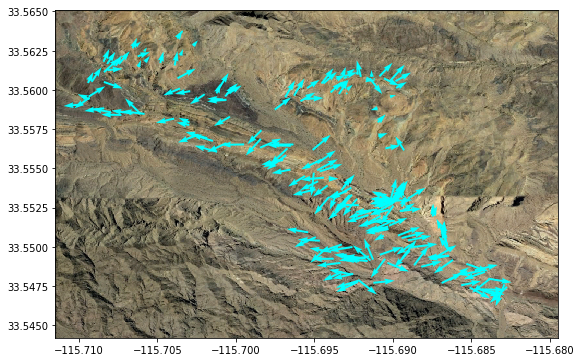This plot tells us an interesting story. Along the center of the satellite image runs a linear feature. To the north of this feature, we see that the arrows are pointing to the north-east. To the south-west of this image, the arrows are pointing south-west. What could be the cause of this pattern?

One probable cause would be a fold or anticline. For an illustration, see the image below. In an anticline, the horizontal layers are tilted away from the axis of the fold, so that the poles to the plane (arrows) are pointing away from the fold axis (dotted line).

In :
Image('Figures/Fold_Diagram.png',width=300)
Out: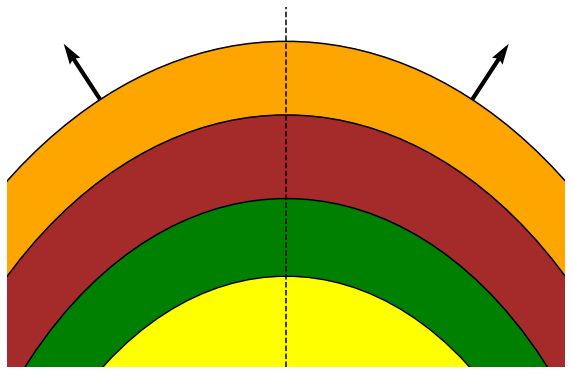Clustering our data¶

Instead of "eyeballing" as we did at first, what if we wanted to automatically sort the two different directions into two different groups? How would we most easily do that? We don't really want to have to train this dataset as we don't really care which group is which in this case, we just want some way of splitting the data into sensible groups. As such we might want to use some kind of unsupervised machine learning process.

The scikit-learn package has a module called sklearn.cluster that allows us to solve this problem. There are many algorithms for different 'shapes' of clusters. Let's try converting our data into a format scikit-learn understands, then use the Kmeans clustering algorithm on them.

Remember from Lecture 16, that scikit-learn requires our data to be in a format in which each datapoint has a set of features which are a bit like coordinates.

In :
input_data=np.array([poles_data.Pole_Az,poles_data.Pole_Plunge]).T
print(input_data[0:5])
[[340.  13.]
[359.  14.]
[347.  11.]
[332.  13.]
[ 12.  32.]]

Now let's do the clustering:

In :
from sklearn.cluster import KMeans
kmeans = KMeans(n_clusters=2) #This tells us that we are using a clustering algorithm with 2 clusters

fit=kmeans.fit(input_data) #Fits the kmeans algorithm to our input data
clusternumbers=kmeans.predict(input_data) #Gives the cluster numbers for each of our clusters
#Plots the equal area with colors for clusters
plot_equal_area(poles_data.Pole_Az,poles_data.Pole_Plunge,colors=clusternumbers)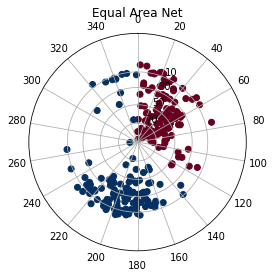Hmm, it seems like this didn't work exactly as expected. Notice how there seems to be a change in cluster across the 0 degree Azimuth line? Let's plot Azimuth against plunge on an x,y plot to see why this didn't seem to work very well.

In :
plt.scatter(poles_data.Pole_Az,poles_data.Pole_Plunge,c=clusternumbers,cmap='RdBu');
plt.xlabel('Azimuth')
plt.ylabel('Plunge');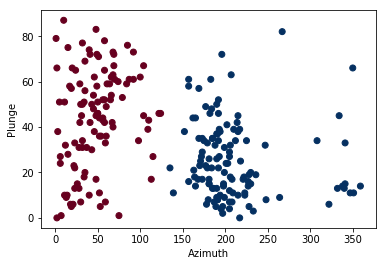The Kmeans algorithm treats data as if they were cartesian. But in geology, we often use directions that go from 0 to 360 which doesn't behave the same way as other cartesian data sets. For example,an azimuth of 340 is closer to 200 than to 0 under this scheme. A simple solution to this would be to convert our azimuths and plunges to cartesian coordinates (as we did for the quiver plot) before clustering. Let's try again:

In :
kmeans = KMeans(n_clusters=2) #This tells us that we are using a clustering algorithm
# with 2 clusters
input_data=np.array([u,v,w]).transpose() # make and array with u,v,w as the first, second and third rows
fit=kmeans.fit(input_data) #Fits the kmeans algorithm to our input data
clusternumbers=kmeans.predict(input_data) #Gives the cluster numbers for each of our clusters
#Plots the equal area with colors for clusters
plot_equal_area(poles_data.Pole_Az,poles_data.Pole_Plunge,colors=clusternumbers)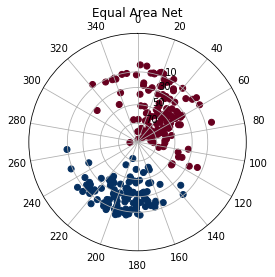Much better! Let's see how it looks on the satellite image!

In :
import matplotlib.pyplot as plt
extent = [-115.7115, -115.6795, 33.5442, 33.5651]